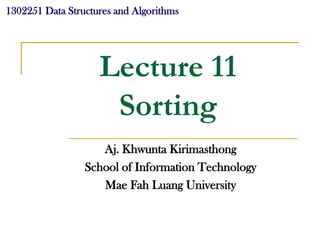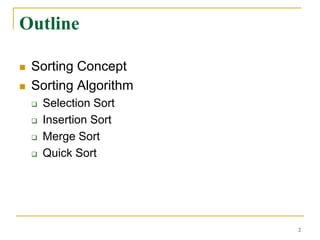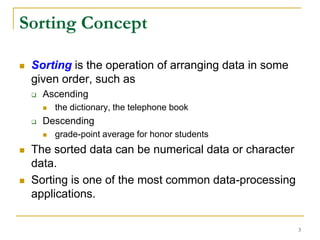Successfully reported this slideshow.

# Lect11 Sorting

2

Share×
1 of 36
1 of 36

# Lect11 Sorting

2

Share

## More Related Content

### Related Books

Free with a 14 day trial from Scribd

See all

### Related Audiobooks

Free with a 14 day trial from Scribd

See all

### Lect11 Sorting

1. 1. 1302251 Data Structures and Algorithms Lecture 11 Sorting Aj. Khwunta Kirimasthong School of Information Technology Mae Fah Luang University
2. 2. Outline  Sorting Concept  Sorting Algorithm  Selection Sort  Insertion Sort  Merge Sort  Quick Sort 2
3. 3. Sorting Concept  Sorting is the operation of arranging data in some given order, such as  Ascending  the dictionary, the telephone book  Descending  grade-point average for honor students  The sorted data can be numerical data or character data.  Sorting is one of the most common data-processing applications. 3
4. 4. Sorting Concept (Cont.)  Sorting problem Let A be a sequence of n elements. Sorting A refers to the operation of rearranging the content of A so that they are increasing in order. Input: A: the sequence of n numbers ( a1 , a 2 , ..., a n ) Output: A: the reordering ( a1 , a 2 ,..., a n ) of the input sequence such that a1 a2 an 4
5. 5. Selection Sort  One of basic sorting algorithms  Selection Sort works as follows:  First, find the smallest elements in the data sequence and exchange it with the element in the first position,  Then, find the second smallest element and exchange it with the element in the second position and  Continue in this way until the entire sequence is sorted. 5
6. 6. Selection Sort Example 1 2 3 4 5 6 1 2 3 4 5 6 A 5 2 4 6 1 3 (1) A 1 2 4 6 5 3 1 2 3 4 5 6 (2) A 1 2 4 6 5 3 1 2 3 4 5 6 (3) A 1 2 3 6 5 4 1 2 3 4 5 6 (4) A 1 2 3 4 5 6 1 2 3 4 5 6 (5) A 1 2 3 4 5 6 1 2 3 4 5 6 (6) A 1 2 3 4 5 6 6
7. 7. Selection Sort Algorithm Algorithm SelectionSort(A,n) Input: A: a sequence of n elements n: the size of A Output: A: a sorted sequence in ascending order for i = 1 to n min = i for j = i+1 to n if A[j] < A[min] then min = j Swap(A,min,i) 7
8. 8. Selection Sort Algorithm (Cont.) Algorithm: Swap(A,min,i) Input: A : a sequence of n elements min: the position of the minimum value of A while considers at index i of A i: the considered index of A Output: exchange A[i] and A[min] temp = a[min] a[min] = a[i] a[i] = temp 8
9. 9. Selection Sort Analysis  For i = 1, there are _(n-1)_ comparisons to find the first smallest element.  For i = 2, there are _(n-2)_ comparisons to find the second smallest element, and so on.  Accordingly, n 1 (n-1) + (n-2) + (n-3) + … + 2 + 1 = i = n(n-1)/2 i 1  The best case running time is ___ (n2)__  The worst case running time is __ O(n2)__ 9
10. 10. Insertion Sort  To solve the sorting problem  Insertion sort algorithm is almost as simple as selection sort but perhaps more flexible.  Insertion sort is the method people often use for sorting a hand of cards. 10
11. 11. Insertion Sort (Cont.)  Insertion sort uses an incremental approach: having the sorted subarray A[1…j-1], we insert the single element A[ j ] into its proper place and then we will get the sorted subarray A[ 1…j ] pick up Sorted order Unsorted order 11
12. 12. Insertion Sort Example 1 2 3 4 5 6 5 2 4 6 1 3 key = A[j] 1 2 3 4 5 6 (1) A 5 2 4 6 1 3 key 2 1 2 3 4 5 6 (2) A 2 5 4 6 1 3 key 4 1 2 3 4 5 6 (3) A 2 4 5 6 1 3 key 6 in-place sorted 12
13. 13. Insertion Sort Example (Cont.) 1 2 3 4 5 6 (4) A 2 4 5 6 1 3 key 1 1 2 3 4 5 6 (5) A 1 2 4 5 6 3 key 3 1 2 3 4 5 6 (6) A 1 2 3 4 5 6 in-place sorted 13
14. 14. Insertion Sort Algorithm Algorithm: INSERTION-SORT(A) Input: A : A sequence of n numbers Output: A : A sorted sequence in increasing order of array A for j = 2 to length(A) key = A[j] //Insert A[j] into the sorted sequence A[1…j-1] i = j-1 while i > 0 and A[i] > key A[i+1] = A[i] i = i-1 A[i+1] = key 14
15. 15. Insertion Sort Analysis  The running – time of INSERTION-SORT depends on the size of input. 15
16. 16. Insertion Sort Analysis (cont.) INSERTION-SORT(A) cost times 1. for j = 2 to length(A) c1 n 2. key = A[j] c2 n-1 3. //Insert A[j] into the sorted sequence A[1…j-1] 0 n-1 4. i = j-1 c4 n-1 n 5. while i > 0 and A[i] > key c5 tj j 2 n 6. A[i+1] = A[i] c6 (t j 1) j 2 n 7. i = i-1 c7 (t j 1) j 2 8. A[i+1] = key c8 n-1 16
17. 17. Analysis of Insertion Sort (Cont.)  To Compute the total running time of INSERTION- SORT, T ( n ) n T (n) c1 n c2 (n 1) 0(n 1) c4 (n 1) c5 tj j 2 n n c6 (t j 1) c7 (t j 1) c8 ( n 1) j 2 j 2 17
18. 18. Analysis of Insertion Sort (Cont.)  Best – case  If input array is already sorted. Thus tj = 1 T(n) = c1n+c2(n-1)+(0)(n-1)+c4(n-1)+c5(n-1)+c6(0)+c7(0)+c8(n-1) = (c1+c2+c3+c4+c5+c8)n - (c2+c3+c4+c5+c8) an + b for constant a and b Base-case running time of INSERTION-SORT is (n) 18
19. 19. Analysis of Insertion Sort (Cont.)  Worse – case  If input array is in reverse sorted order. Thus tj = j n(n 1) T (n) c1 n c2 (n 1) c4 (n 1) c5 1 2 (n 1) n (n 1) n c6 c7 c8 ( n 1) 2 2 c5 c6 c6 2 c5 c6 c7 T (n) n c1 c2 c4 c8 n 2 2 2 2 2 2 (c2 c4 c5 c8 ) an 2 bn c for constant a, b and c 2 Worse-case running time of INSERTION-SORT is (n ) 19
20. 20. Analysis of Insertion Sort (Cont.)  Worse – case (Cont.)  If input array is in reverse sorted order. Thus tj = j n n n n(n 1) n(n 1) j tj j 1 j 1 2 j 2 j 2 2 n n (n 1)(( n 1) 1) (n 1) n (t j 1) (j 1) (1 1) j 2 j 2 2 2 20
21. 21. Divide and Conquer Approach Merge Sort Quick Sort
22. 22. Divide-and-Conquer Approach  The divide-and-conquer approach involves three steps at each level of the recursion:  Divide the problem into a number of sub-problems that are similar to the original problem but smaller in size.  Conquer the sub-problems by solving them recursively.  Combine the solution of subproblems into the solution for the original problem. 22
23. 23. Merge Sort  The Merge Sort algorithm closely follows the divide-and-conquer approach. It operates as follows,  Divide: Divide the n-element sequence to be sorted into two subsequences of n/2 elements each.  Conquer: Sort the two subsequences recursively using merge sort  Combine: Merge the two sorted subsequences to produce the sorted solution. 23
24. 24. Merge Sort (Cont.)  The recursion “bottom out” when the sequence to be sorted has length 1, in which case there is no work to be done, since every sequence of length 1 is already in sorted order. 24
25. 25. Merge Sort Example DIVIDE & 0 1 2 3 4 5 CONQUER A 5 2 4 6 1 3 0 1 2 3 4 5 5 2 4 6 1 3 0 1 2 3 4 5 5 2 4 6 1 3 0 1 3 4 5 2 6 1 25
26. 26. Merge Sort Example (cont.) 0 1 2 3 4 5 COMBINE A 1 2 3 4 5 6 0 1 2 3 4 5 2 4 5 1 3 6 0 1 2 3 4 5 2 5 4 1 6 3 0 1 3 4 5 2 6 1 26
27. 27. Merge Sort Algorithm Algorithm MergeSort( A, p, q ) Input: A: a sequence of n elements p: the beginning index of A q: the last index of A Output: A: a sorted sequence of n elements if(p < q) then r = floor((p+q)/2) MergeSort(A,p,r) MergeSort(A,r+1,q) Merge(A,p,r,q) 27
28. 28. Algo Merge(A,p,r,q) Input: A, p, r, q : a sorted subsequences A[p…r] and A[r+1…q] Output: A: a sorted sequence A[p…q] let i=p, k=p, j=r+1 while (i ≤ r) and (j ≤ q) if (A[i] ≤ A[j]) then B[k] = A[i] k++ i++ else B[k] = A[j] k=k+1 j=j+1 if (i>r) then /* If the maximum value of left subsequence is less than the right subsequence */ while(j ≤ q) B[k] = A[j] k++ j++ else if(j > q) then /* If the maximum value of left subsequence is gather than the right subsequence */ while(i ≤ r) B[k] = A[i] k++ i++ for k = p to q A[k] = b[k] 28
29. 29. Merge Sort Analysis  The best case running time is _ (n log n)_  The worst case running time is _ O(n log n) _ 29
30. 30. Quick Sort  Quick Sort, likes merge sort, is based on the divide-and-conquer approach.  To sort an array A[p…r]  Divide: Partition (rearrange) the array A[p…r] into two subarray A[p…q -1] and subarray A[q+1…r] such that:  Each element of A[p…q -1] is less than or equal to A[q]  Each element of A[q+1…r] is greater than A[q] 30
31. 31. Quick Sort (Cont.)  To sort sorting an array A[p…r] (cont.)  Conquer: Sort the two subarray A[p…q -1] and A[q+1…r] by recursive calls to QuickSort.  Combine: Since the subarrays are sorted in place, no work is needed to combine them: the entire array A[p…r] is now sorted. 31
32. 32. Quick Sort Example 0 1 2 3 4 5 A 5 2 4 6 1 3 32
33. 33. Quick Sort Algorithm Algo QuickSort(A, p, r) Input: A: A sequence of n numbers p: The first index of A r: The last index of A Output: A: A sorted sequence in increasing order of array A • Rearrange the subarray if (p < r) then A[p…r] in place. • The elements that is less q = Partition(A,p,r) than A[q] are placed at the left side of A[q] and QuickSort(A,p,q-1) • The elements that is greater than A[q] are placed QuickSort(A,q+1,r) at the right side of A[q]. 33
34. 34. Quick Sort Algorithm (Cont.) Algo Partition(A, p, r) Input: A: A sequence of n numbers p: The first index of A r: The last index of A Output: A: The rearranged subarray A[p…r] key = A[r] i=p–1 for j = p to r -1 if A[ j ] ≤ key then i = i+1 exchange A[i] and A[j] exchange A[i+1] and key return i+1 34
35. 35. Quick Sort Analysis  The best case running time is _ (n log n)_  The worst case running time is _ O(n2)_ 35
36. 36. Q&A